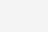# 函数式编程

• 函数式编程的三大特性：

• immutable data 不可变数据：像Clojure一样，默认上变量是不可变的，如果你要改变变量，你需要把变量copy出去修改。这样一来，可以让你的程序少很多Bug。因为，程序中的状态不好维护，在并发的时候更不好维护。（你可以试想一下如果你的程序有个复杂的状态，当以后别人改你代码的时候，是很容易出bug的，在并行中这样的问题就更多了）

• first class functions：这个技术可以让你的函数就像变量一样来使用。也就是说，你的函数可以像变量一样被创建，修改，并当成变量一样传递，返回或是在函数中嵌套函数。这个有点像Javascript的Prototype（参看Javascript的面向对象编程

• 尾递归优化：我们知道递归的害处，那就是如果递归很深的话，stack受不了，并会导致性能大幅度下降。所以，我们使用尾递归优化技术——每次递归时都会重用stack，这样一来能够提升性能，当然，这需要语言或编译器的支持。Python就不支持。

• 函数式编程的几个技术

• map & reduce ：这个技术不用多说了，函数式编程最常见的技术就是对一个集合做Map和Reduce操作。这比起过程式的语言来说，在代码上要更容易阅读。（传统过程式的语言需要使用for/while循环，然后在各种变量中把数据倒过来倒过去的）这个很像C++中的STL中的foreach，find_if，count_if之流的函数的玩法。

• pipeline：这个技术的意思是，把函数实例成一个一个的action，然后，把一组action放到一个数组或是列表中，然后把数据传给这个action list，数据就像一个pipeline一样顺序地被各个函数所操作，最终得到我们想要的结果。

• recursing 递归 ：递归最大的好处就简化代码，他可以把一个复杂的问题用很简单的代码描述出来。注意：递归的精髓是描述问题，而这正是函数式编程的精髓。

• currying：把一个函数的多个参数分解成多个函数， 然后把函数多层封装起来，每层函数都返回一个函数去接收下一个参数这样，可以简化函数的多个参数。在C++中，这个很像STL中的bind_1st或是bind2nd。

• higher order function 高阶函数：所谓高阶函数就是函数当参数，把传入的函数做一个封装，然后返回这个封装函数。现象上就是函数传进传出，就像面向对象对象满天飞一样。

• 还有函数式的一些好处

• parallelization 并行：所谓并行的意思就是在并行环境下，各个线程之间不需要同步或互斥。

• lazy evaluation 惰性求值：这个需要编译器的支持。表达式不在它被绑定到变量之后就立即求值，而是在该值被取用的时候求值，也就是说，语句如x:=expression; (把一个表达式的结果赋值给一个变量)明显的调用这个表达式被计算并把结果放置到 x 中，但是先不管实际在 x 中的是什么，直到通过后面的表达式中到 x 的引用而有了对它的值的需求的时候，而后面表达式自身的求值也可以被延迟，最终为了生成让外界看到的某个符号而计算这个快速增长的依赖树。

• determinism 确定性：所谓确定性的意思就是像数学那样 f(x) = y ，这个函数无论在什么场景下，都会得到同样的结果，这个我们称之为函数的确定性。而不是像程序中的很多函数那样，同一个参数，却会在不同的场景下计算出不同的结果。所谓不同的场景的意思就是我们的函数会根据一些运行中的状态信息的不同而发生变化。

```int cnt;
void increment(){
cnt++;
}```

```int increment(int cnt){
return cnt+1;
}```

```def inc(x):
def incx(y):
return x+y
return incx
inc2 = inc(2)
inc5 = inc(5)
print inc2(5) # 输出 7
print inc5(5) # 输出 10```

#### Map & Reduce

``` name_len = map(len, ["hao", "chen", "coolshell"])
print name_len
# 输出 [3, 4, 9]```

```def toUpper(item):
return item.upper()
upper_name = map(toUpper, ["hao", "chen", "coolshell"])
print upper_name
# 输出 ['HAO', 'CHEN', 'COOLSHELL']```

```#include <iostream>
#include <algorithm>
#include <string>
using namespace std;
int main() {
string s="hello";
string out;
transform(s.begin(), s.end(), back_inserter(out), ::toupper);
cout << out << endl;
// 输出：HELLO
}```

```upname =['HAO', 'CHEN', 'COOLSHELL']
lowname =[]
for i in range(len(upname)):
lowname.append( upname[i].lower() )```

```squares = map(lambda x: x * x, range(9))
print squares
# 输出 [0, 1, 4, 9, 16, 25, 36, 49, 64]```

```print reduce(lambda x, y: x+y, [1, 2, 3, 4, 5])
# 输出 15```

Python中的除了map和reduce外，还有一些别的如filter, find, all, any的函数做辅助（其它函数式的语言也有），可以让你的代码更简洁，更易读。 我们再来看一个比较复杂的例子：

```num =[2, -5, 9, 7, -2, 5, 3, 1, 0, -3, 8]
positive_num_cnt = 0
positive_num_sum = 0
for i in range(len(num)):
if num[i] > 0:
positive_num_cnt += 1
positive_num_sum += num[i]
if positive_num_cnt > 0:
average = positive_num_sum / positive_num_cnt
print average
# 输出 5```

```positive_num = filter(lambda x: x>0, num)
average = reduce(lambda x,y: x+y, positive_num) / len( positive_num )```

C++11玩的法：

```#include <iostream>
#include <algorithm>
#include <numeric>
#include <string>
#include <vector>
using namespace std;
vector num {2, -5, 9, 7, -2, 5, 3, 1, 0, -3, 8};
vector p_num;
copy_if(num.begin(), num.end(), back_inserter(p_num), [](int i){ return (i>0);} );
int average = accumulate(p_num.begin(), p_num.end(), 0) / p_num.size();
cout << "averge: " << average << endl;```

1）代码更简单了。
2）数据集，操作，返回值都放到了一起。
3）你在读代码的时候，没有了循环体，于是就可以少了些临时变量，以及变量倒来倒去逻辑。
4）你的代码变成了在描述你要干什么，而不是怎么去干。

```var map = function (mappingFunction, list) {
var result = [];
forEach(list, function (item) {
result.push(mappingFunction(item));
});
return result;
};```

```function reduce(actionFunction, list, initial){
var accumulate;
var temp;
if(initial){
accumulate = initial;
}
else{
accumulate = list.shfit();
}
temp = list.shift();
while(temp){
accumulate = actionFunction(accumulate,temp);
temp = list.shift();
}
return accumulate;```

```from random import random
time = 5
car_positions = [1, 1, 1]
while time:
# decrease time
time -= 1
print ''
for i in range(len(car_positions)):
# move car
if random() > 0.3:
car_positions[i] += 1
# draw car
print '-' * car_positions[i # draw car
print '-' * car_positions[i]]```

```from random import random

def move_cars():
for i, _ in enumerate(car_positions):
if random() > 0.3:
car_positions[i] += 1

def draw_car(car_position):
print '-' * car_position

def run_step_of_race():
global time
time -= 1
move_cars()

def draw():
print ''
for car_position in car_positions:
draw_car(car_position)

time = 5
car_positions = [1, 1, 1]

while time:
run_step_of_race()
draw()```

```from random import random

def move_cars(car_positions):
return map(lambda x: x + 1 if random() > 0.3 else x,
car_positions)

def output_car(car_position):
return '-' * car_position

def run_step_of_race(state):
return {'time': state['time'] - 1,
'car_positions': move_cars(state['car_positions'])}

def draw(state):
print ''
print '\n'.join(map(output_car, state['car_positions']))

def race(state):
draw(state)
if state['time']:
race(run_step_of_race(state))

race({'time': 5,
'car_positions': [1, 1, 1]})```

1）它们之间没有共享的变量。
2）函数间通过参数和返回值来传递数据。
3）在函数里没有临时变量。#### Pipeline

pipeline 管道借鉴于Unix Shell的管道操作——把若干个命令串起来，前面命令的输出成为后面命令的输入，如此完成一个流式计算。（注：管道绝对是一个伟大的发明，他的设哲学就是KISS – 让每个功能就做一件事，并把这件事做到极致，软件或程序的拼装会变得更为简单和直观。这个设计理念影响非常深远，包括今天的Web Service，云计算，以及大数据的流式计算等等）

`ps auwwx | awk '{print \$2}' | sort -n | xargs echo`

`xargs(  echo, sort(n, awk('print \$2', ps(auwwx)))  )`

`pids = for_each(result, [ps_auwwx, awk_p2, sort_n, xargs_echo])`

1）找出偶数。
2）乘以3
3）转成字符串返回

```def process(num):
# filter out non-evens
if num % 2 != 0:
return
num = num * 3
num = 'The Number: %s' % num
return num

nums = [1, 2, 3, 4, 5, 6, 7, 8, 9, 10]

for num in nums:
print process(num)

# 输出：
# None
# The Number: 6
# None
# The Number: 12
# None
# The Number: 18
# None
# The Number: 24
# None
# The Number: 30```

```def even_filter(nums):
for num in nums:
if num % 2 == 0:
yield num
def multiply_by_three(nums):
for num in nums:
yield num * 3
def convert_to_string(nums):
for num in nums:
yield 'The Number: %s' % num

nums = [1, 2, 3, 4, 5, 6, 7, 8, 9, 10]
pipeline = convert_to_string(multiply_by_three(even_filter(nums)))
for num in pipeline:
print num
# 输出：
# The Number: 6
# The Number: 12
# The Number: 18
# The Number: 24
# The Number: 30```

```def even_filter(nums):
return filter(lambda x: x%2==0, nums)
def multiply_by_three(nums):
return map(lambda x: x*3, nums)
def convert_to_string(nums):
return map(lambda x: 'The Number: %s' % x,  nums)
nums = [1, 2, 3, 4, 5, 6, 7, 8, 9, 10]
pipeline = convert_to_string(
multiply_by_three(
even_filter(nums)
)
)
for num in pipeline:
print num```

```pipeline_func(nums, [even_filter,
multiply_by_three,
convert_to_string])```

```def pipeline_func(data, fns):
return reduce(lambda a, x: x(a),
fns,
data)```

#### 参考

```class Pipe(object):
def __init__(self, func):
self.func = func
def __ror__(self, other):
def generator():
for obj in other:
if obj is not None:
yield self.func(obj)
return generator()
@Pipe
def even_filter(num):
return num if num % 2 == 0 else None
@Pipe
def multiply_by_three(num):
return num*3
@Pipe
def convert_to_string(num):
return 'The Number: %s' % num
@Pipe
def echo(item):
print item
return item
def force(sqs):
for item in sqs: pass
nums = [1, 2, 3, 4, 5, 6, 7, 8, 9, 10]
force(nums | even_filter | multiply_by_three | convert_to_string | echo)```

（全文完）

(0)

### 相关推荐

• #### N22-第一周练习作业-冥界之王

1.描述计算机系统的组成及功能硬件组成(输入设备,输出设备,存储器,运算器,控制器) 输入设备：鼠标，键盘，麦克，声卡（输入声音），扫描仪，手写板等输出设备：显示器，声卡（输出声音），音箱，投影仪等存储器：内存，硬盘，磁带，CPU内部还可以分1，2，3级缓存运算器：主要完成对二进制数据的定点算术运算（加减乘除）、逻辑运算（与或非异或）以及移位操作。在某些CP…

Linux干货 2016-08-15
• #### 关于生命中的根

关于生命中的根    擦干心中的血和泪痕    留住我们的根 关于生命中的根 鸿蒙初辟,万物生于根 寻根之旅 根就是根 我即为根 路在何方 鸿蒙初辟,万物生于根    自linux老祖Torvalds创根之始至今,根一直为linux之本,而FHS(Filesystem Hierarchy Stand…

Linux干货 2016-10-27
• #### shell编程之数组及环境变量

Linux干货 2016-08-29
• #### 第三周作业

1、列出当前系统上所有已登陆的用户名：          [root@yangjifeng~]# who | cut -d ‘ ‘ -f 1 | sort -u     root 2、取出最后登陆到当前系统的用户信息； &nb…

Linux干货 2017-08-28
• #### 文件、目录2——Linux基本命令（8）

1.复制文件和目录 cp  SRC  DEST 源 目的地 规则： 如果目标目录下还有一个目录与源文件同名，则无法复制：            -i 覆盖前提示        &n…

2017-07-18
• #### Nginx 编译安装

Nginx (“engine x”) 是一个高性能的 HTTP 和 反向代理服务器，也是一个 IMAP/POP3/SMTP 代理服 1、Apache服务器和nginx的优缺点： Apache具有很优秀的性能，而且通过模块可以提供各种丰富的功能。 1)首先Apache对客户端的响应是支持并发的 ，运行httpd这个daemon进程之后，它会同时产生多个子进程/…

Linux干货 2017-02-06